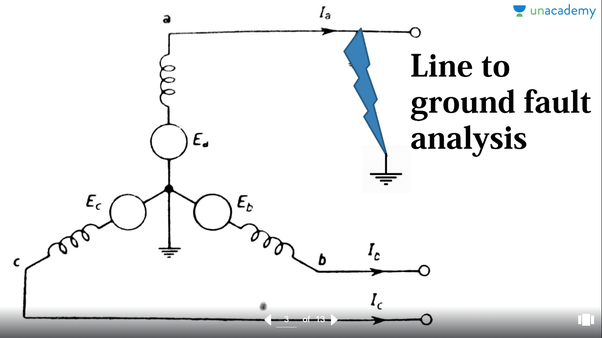Photo Source: UNACADEMY

In three phase system, Single Line to Ground Fault happens when one of the lines or phase of the system is accidentally connected to ground. In this case unbalanced voltage will be developed in the system as well as unbalanced current.

See Sample of Single Line to Ground Fault Calculation

By principle, all sequence components i.e. positive, negative and zero sequence components will be connected in series. See article: How to Develop Sequence Network in an Unbalanced System?

In this regard, the above diagram can be further represented below.Single Line to Ground Fault

From the figure above, we can see that the fault current is equal to Ia. On the other hand, Ib and Ic will be both zero since there will be no fault current that will flow from that phases.

Thus the fault currents in this case can be summarize as:
• Fault Current at A = Ia
• Fault Current at B = 0
• Fault Current at C = 0
Using the principle of symmetrical components we can get the corresponding sequence values. (See article: What is Symmetrical Components?)

• I0 = IA
• I1 = IA
• I2 = IA
Therefore we can present the sequence network as follows:

In this case, all sequence component networks i.e. the positive, negative and zero sequence are connected in series. This will affirm the equality of all sequence currents.

See Sample of Single Line to Ground Fault Calculation